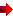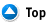College MathematicsMathematics Mathematics Calculus: Introduction Calculus: Applied Math: Statistics Any College Math

Mathematics 1

# Mathematics

This course covers basic numerical computations and operations with exact and approximate numbers; basic algebraic operations and simple linear equations; factoring techniques; operations with algebraic fraction; fractional equations and formula rearrangement; functional notation and graphing; systems of linear equations (2 equations, 2 variables); quadratic equations; variation (direct, inverse, joint) trigonometric concepts including angle measure (degrees and radians), arc length problems, basic trigonometric ratios, right triangle solutions, trigonometric ratios of any angle, basic trig. functions, laws of sines and cosines, vector problems.

## Mathematics

Systems of 3 linear equations in 3 unknowns; graphs of the trigonometric functions; J-operator; use of semi-log and log-log graph paper; additional types of equations and systems of equations; progressions and the Binomial theorem; additional topics in trigonometry and analytic geometry.

Prerequisites: Mathematics 1

Calculus: Introduction

This mathematics course covers limits and derivatives; derivatives of algebraic functions; rates of change and optimization problems; derivatives of trigonometric, logarithmic and exponential functions; simple integration; indefinite and definite integrals; applications of the integral; partial derivatives; applications of partial differentiation.

Prerequisites: Mathematics 1/Mathematics 2

Calculus: Applied

This mathematics course covers review of differentiation and integration, integration methods, first and second order differential equations, laplace transforms, infinite series (Maclaurin, Taylor, Fourier), and applications.

Prerequisites: Calculus: Introduction

### Math: Statistics

The course provides an introduction to statistical theory and techniques with direct applications to established industrial practices of quality assurance and process control.

Prerequisite: Mathematics 1 or Mathematics 2Register ON-LINE